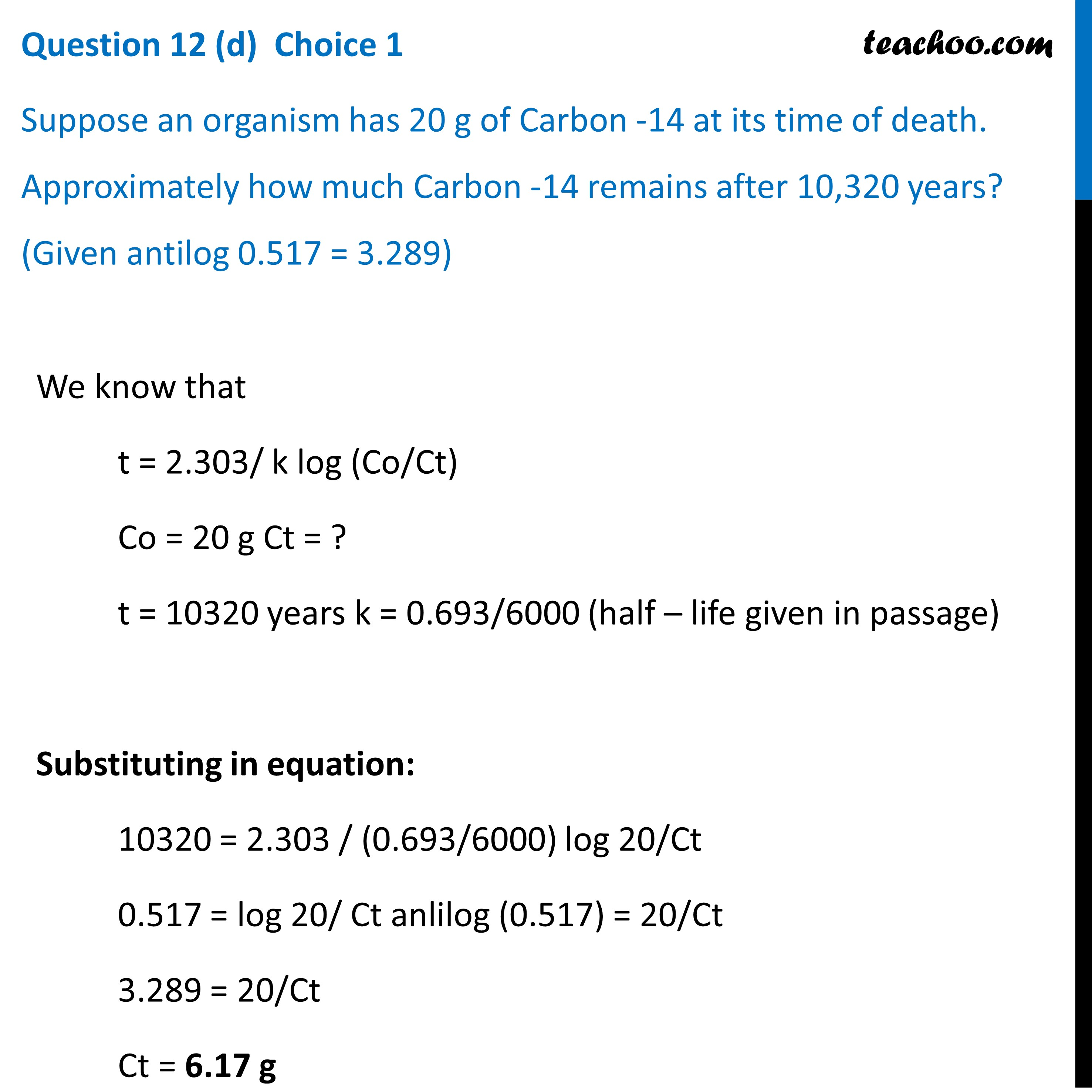Chemistry CBSE Class 12 Sample Paper for 2022 Boards [Term 2]

Chemistry Class 12
Solutions to CBSE Sample Paper - Chemistry Class 12

## Suppose an organism has 20 g of Carbon -14 at its time of death. Approximately how much Carbon -14 remains after 10,320 years? (Given antilog 0.517 = 3.289)

(d)t = 2.303/ k log (Co/Ct)

Co = 20 g Ct = ?

t = 10320 years k = 0.693/6000 (half-life given in passage)

substituting in equation:

10320 = 2.303 / (0.693/6000) log 20/ Ct

0.517 = log 20 / Ct anlilog (0.517) = 20/Ct3.289 = 20/Ct

Ct = 6.17 g

Learn in your speed, with individual attention - Teachoo Maths 1-on-1 Class